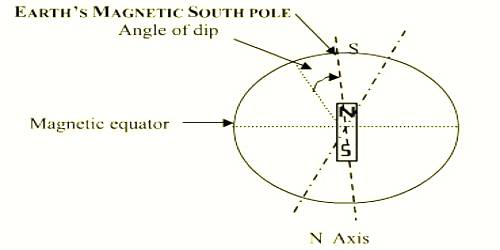# Angle of dip or inclination: Elements of Geomagnetism

The angle of dip or inclination: Elements of Geomagnetism

An angle of Dip or inclination is the angle made with the horizontal by the Earth’s magnetic field lines. A wooden rod suspended by a torsion-free thread from its center of gravity always remains horizontal (Figure). But a magnetic needle or a bar magnet similarly suspended will not come to rest in a horizontal position but will make some angle with the horizontal plane (Figure). This angle is called the angle of clip or inclination.Definition: The angle between the direction of the earth’s magnetic field at a place and the horizontal direction measured in the plane of the magnetic meridian is called the angle of dip of the geomagnetism of that place. It is denoted by δ.

The angle of Dip (δ) is the angle in the vertical plane aligned with the magnetic north (the magnetic meridian) between the local magnetic field and the horizontal. The angle of dip is different at different places of the earth. If a suspended bar magnet is gradually taken towards the northern hemisphere, the north pole of the magnet will be found to dip more and more making a gradual increase of angle with the horizontal downwards and in that case, the angle of dip is written as δ°N or δ0 North or δ0 N. Again in the southern hemisphere the south pole of the magnet will dip downwards. In these positions, the angle of dip is written as δ°S or δ0 south or δ0 S. In the two poles dip is 90° and at the equator, it is 00.

The magnetic poles are defined as the area where the dip is vertical. You can compute this area using magnetic field models, such as the World Magnetic Model (WMM) and the International Geomagnetic Reference Field (IGRF). You can also survey for the magnetic pole, using instruments that measure the magnetic field strength and direction.

Now the question arises why the magnetic axis of a freely suspended magnet does not remain horizontal other than at taw equation? We know, the earth is a huge magnet. So, there is a direction of the geomagnetic field intensity. It remains inclined with the horizontal other than that at the equator. Since freely suspended magnet aligns itself along the direction of the geomagnetic field intensity, hence the suspended magnet without remaining in the horizontal makes some angle with it.

Example: “Angle of dip of Dhaka is 31°N” means that if a bar magnet is suspended freely from its center of gravity, the north pole of the magnetic will remain hanging downward, and its magnetic axis will make an angle of 31° with the horizontal.

The strength of the Earth’s magnetic field varies like that of a bar magnet, strongest at the poles and weakest at the equator. In addition, the angles of magnetic flux lines vary across the surface of the Earth, vertical at the magnetic poles and tangential to the Earth’s surface at the magnetic equator. Consequently, the angle of the magnetic flux lines is correlated with the strength of the magnetic field.